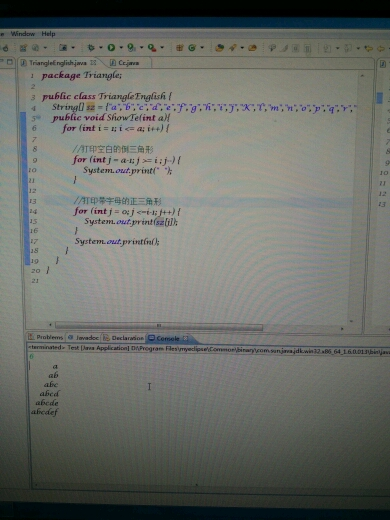###### big_bai

2016-05-25 02:13 阅读 6.6k

# java数组的倒序输出。

1

a
ba
cba
dcba
…………

• 点赞
• 写回答
• 关注问题
• 收藏
• 复制链接分享

#### 5条回答默认 最新

• 已采纳
`````` /* package whatever; // don't place package name! */

import java.util.*;
import java.lang.*;
import java.io.*;

/* Name of the class has to be "Main" only if the class is public. */
class Ideone
{
static String[] sz = { "a", "b", "c", "d", "e", "f" /* ... */ };

static void showTable(int n)
{
for (int i = 1; i <= n; i++)
{
for (int j = 0; j <= n - i; j++)
{
System.out.print(" ");
}
for (int j = i - 1; j >= 0; j--)
{
System.out.print(sz[j]);
}
System.out.println();
}
}
public static void main (String[] args) throws java.lang.Exception
{
// your code goes here
showTable(5);
}
}
``````
点赞 评论 复制链接分享
• `````` String s = "abcde";
String s1 = "";
for (int i = s.lengh() - 1; i >= 0; i--)
s1 += s.charAt(i);
System.out.println(s1);
``````
点赞 评论 复制链接分享
• for (char M = 'Z'; M >= 'A'; M--) {
System.out.print(M);
}

点赞 评论 复制链接分享
• 把打印的内容变为
a
ba
cba
dcba
edcba
.………………
这样的。点赞 评论 复制链接分享
• http://ideone.com/J9LhfP

在线输出结果

点赞 评论 复制链接分享